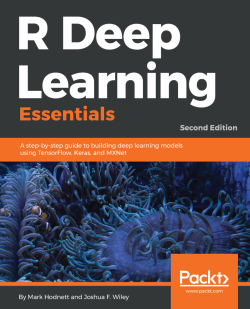# R Deep Learning Essentials - Second Edition4 (1 reviews total)
By Mark Hodnett , Joshua F. Wiley
• \$33.99 eBook
• \$41.99 Print + eBook
• \$12.99 eBook + Subscription
What do you get with a Packt Subscription?

• Constantly updated with 100+ new titles each month
• Breadth and depth in over 1,000+ technologies

Deep learning is a powerful subset of machine learning that is very successful in domains such as computer vision and natural language processing (NLP). This second edition of R Deep Learning Essentials will open the gates for you to enter the world of neural networks by building powerful deep learning models using the R ecosystem.

This book will introduce you to the basic principles of deep learning and teach you to build a neural network model from scratch. As you make your way through the book, you will explore deep learning libraries, such as Keras, MXNet, and TensorFlow, and create interesting deep learning models for a variety of tasks and problems, including structured data, computer vision, text data, anomaly detection, and recommendation systems. You’ll cover advanced topics, such as generative adversarial networks (GANs), transfer learning, and large-scale deep learning in the cloud. In the concluding chapters, you will learn about the theoretical concepts of deep learning projects, such as model optimization, overfitting, and data augmentation, together with other advanced topics.

By the end of this book, you will be fully prepared and able to implement deep learning concepts in your research work or projects.Use R 3.5 for building deep learning models for computer vision and text

Publication date:
August 2018
Publisher
Packt
Pages
378
ISBN
9781788992893

# Getting Started with Deep Learning

This chapter discusses deep learning, a powerful multilayered architecture for pattern-recognition, signal-detection, and classification or prediction. Although deep learning is not new, it is only in the past decade that it has gained great popularity, due in part to advances in computational capacity and new ways of more efficiently training models, as well as the availability of ever-increasing amounts of data. In this chapter, you will learn what deep learning is, the R packages available for training such models, and how to get your system set up for analysis. We will briefly discuss MXNet and Keras, which are the two main frameworks that we will use for many of the examples in later chapters to actually train and use deep learning models.

In this chapter, we will explore the following topics:

• What is deep learning?
• A conceptual overview of deep learning
• Setting up your R environment and the deep learning frameworks available in R
• GPUs and reproducibility

# What is deep learning?

Deep learning is a subfield within machine learning, which in turn is a subfield within artificial intelligence. Artificial intelligence is the art of creating machines that perform functions that require intelligence when performed by people. Machine learning uses algorithms that learn without being explicitly programmed. Deep learning is the subset of machine learning that uses artificial neural networks that mimic how the brain works.

The following diagram shows the relationships between them. For example, self-driving cars are an application of artificial intelligence. A critical part of self-driving cars is to recognize other road users, cars, pedestrians, cyclists, and so on. This requires machine learning because it is not possible to explicitly program this. Finally, deep learning may be chosen as the method to implement this machine learning task: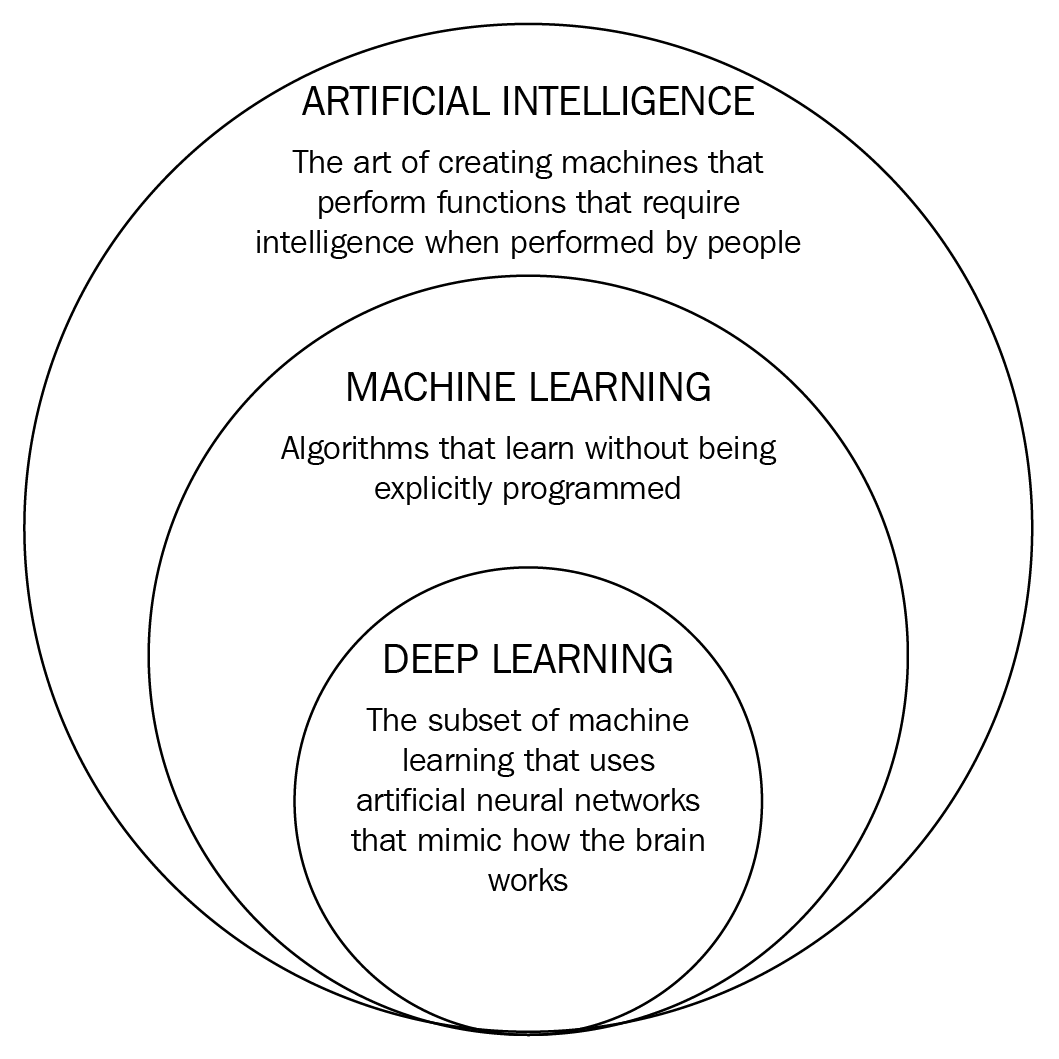Figure 1.1: The relationship between artificial intelligence, machine learning, and deep learning

Artificial intelligence as a field has existed since the 1940s; the definition used in the previous diagram is from Kurzweil, 1990. It is a broad field that encompasses ideas from many different fields, including philosophy, mathematics, neuroscience, and computer engineering. Machine learning is a subfield within artificial intelligence that is devoted to developing and using algorithms that learn from raw data. When the machine learning task has to predict an outcome, it is known as supervised learning. When the task is to predict from a set of possible outcomes, it is a classification task, and when the task is to predict a numeric value, it is a regression task. Some examples of classification tasks are whether a particular credit card purchase is fraudulent, or whether a given image is of a cat or a dog. An example of a regression task is predicting how much money a customer will spend in the next month. There are other types of machine learning where the learning does not predict values. This is called unsupervised learning and includes clustering (segmenting) the data, or creating a compressed format of the data.

Deep learning is a subfield within machine learning. It is called deep because it uses multiple layers to map the relationship between input and output. A layer is a collection of neurons that perform a mathematical operation on its input. This will be explained in more detail in the next section, Conceptual overview of neural networks. This deep architecture means the model is large enough to handle many variables and that it is sufficiently flexible to approximate the patterns in the data. Deep learning can also generate features as part of the overall learning algorithm, rather than feature-creation being a prerequisite step. Deep learning has proven particularly effective in the fields of image-recognition (including handwriting as well as photo- or object-classification) , speech recognition and natural-language. It has completely transformed how to use image, text, and speech data for prediction in the past few years, replacing previous methods of working with these types of data. It has also opened up these fields to a lot more people because it automates a lot of the feature-generation, which required specialist skills.

Deep learning is not the only technique available in machine learning. There are other types of machine learning algorithms; the most popular include regression, decision trees, random forest, and naive bayes. For many use cases, one of these algorithms could be a better choice. Some examples of use cases where deep learning may not be the best choice include when interpretability is an essential requirement, the dataset size is small, or you have limited resources (time and/or hardware) to develop a model. It is important to realize that despite, the industry hype, most machine learning in industry does not use deep learning. Having said that, this book covers deep learning algorithms, so we will move on. The next sections will discuss neural networks and deep neural networks in more depth.

# A conceptual overview of neural networks

It can be difficult to understand why neural networks work so well. This introduction will look at them from two viewpoints. If you have an understanding of how linear regression works, the first viewpoint should be useful. The second viewpoint is more intuitive and less technical, but equally valid. I encourage you to read both and spend some time contemplating both overviews.

# Neural networks as an extension of linear regression

One of the simplest and oldest prediction models is regression. It predicts a continuous value (that is, a number) based on another value. The linear regression function is:

y=mx+b

Where y is the value you want to predict and x is your input variable. The linear regression coefficients (or parameters) are m (the slope of the line) and b (the intercept). The following R code creates a line with the y= 1.4x -2 function and plots it:

`set.seed(42)m <- 1.4b <- -1.2x <- 0:9jitter<-0.6xline <- xy <- m*x+bx <- x+rnorm(10)*jittertitle <- paste("y = ",m,"x ",b,sep="")plot(xline,y,type="l",lty=2,col="red",main=title,xlim=c(0,max(y)),ylim=c(0,max(y)))points(x[seq(1,10,2)],y[seq(1,10,2)],pch=1)points(x[seq(2,11,2)],y[seq(2,11,2)],pch=4)`

The o or x points are the values to be predicted given a value on the x axis and the line is the ground truth. Some random noise is added, so that the points are not exactly on the line. This code produces the following output: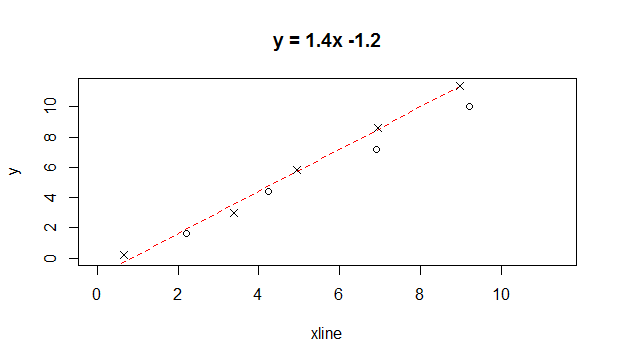Figure 1.2: Example of a regression line fitted to data (that is, predict y from x)

In a regression task, you are given some x and corresponding y values, but are not given the underlying function to map x to y. The purpose of a supervised machine learning task is that given some previous examples of x and y, can we predict the y values for new data where we only have x and not y. An example might be to predict house prices based on the number of bedrooms in the house. So far, we have only considered a single input variable, x, but we can easily extend the example to handle multiple input variables. For the house example, we would use the number of bedrooms and square footage to predict the price of the house. Our code can accommodate this by changing the input, x, from a vector (one-dimensional array) into a matrix (two-dimensional array).

If we consider our model for predicting house prices, linear regression has a serious limitation: it can only estimate linear functions. If the mapping from x to y is not linear, it will not predict y very well. The function always results in a straight line for one variable and a hyperplane if multiple x predictor values are used. This means that linear regression models may not be accurate at the low and high extent of the data.

A simple trick to make the model fit nonlinear relationships is to add polynomial terms to the function. This is known as polynomial regression. For example, by adding a polynomial of degree 4, our function changes to:

y= m1x4+ m2x3+ m3x2+ m4x+b

By adding these extra terms, the line (or decision boundary) is no longer linear. The following code demonstrates this we create some sample data and we create three regression models to fit this data. The first model has no polynomial terms, the model is a straight line and fits the data very poorly. The second model (blue circles) has polynomials up to degree 3, that is, X, X2, and X3. The last model has polynomials up to degree 12, that is, X, X2,....., X12. The first model (straight line) underfits the data and the last line overfits the data. Overfitting refers to situations where the model is too complex and ends up memorizing the data. This means that the model does not generalize well and will perform poorly on unseen data. The following code generates the data and creates three models with increasing levels of polynomial:

`par(mfrow=c(1,2))set.seed(1)x1 <- seq(-2,2,0.5) # y=x^2-6jitter<-0.3y1 <- (x1^2)-6x1 <- x1+rnorm(length(x1))*jitterplot(x1,y1,xlim=c(-8,12),ylim=c(-8,10),pch=1)x <- x1y <- y1# y=-xjitter<-0.8x2 <- seq(-7,-5,0.4)y2 <- -x2x2 <- x2+rnorm(length(x2))*jitterpoints(x2,y2,pch=2)x <- c(x,x2)y <- c(y,y2)# y=0.4 *rnorm(length(x3))*jitterjitter<-1.2x3 <- seq(5,9,0.5)y3 <- 0.4 *rnorm(length(x3))*jitterpoints(x3,y3,pch=3)x <- c(x,x3)y <- c(y,y3) df <- data.frame(cbind(x,y))plot(x,y,xlim=c(-8,12),ylim=c(-8,10),pch=4)model1 <- lm(y~.,data=df)abline(coef(model1),lty=2,col="red")max_degree<-3for (i in 2:max_degree){ col<-paste("x",i,sep="") df[,col] <- df\$x^i}model2 <- lm(y~.,data=df)xplot <- seq(-8,12,0.1)yplot <- (xplot^0)*model2\$coefficientsfor (i in 1:max_degree) yplot <- yplot +(xplot^i)*model2\$coefficients[i+1]points(xplot,yplot,col="blue", cex=0.5) max_degree<-12for (i in 2:max_degree){ col<-paste("x",i,sep="") df[,col] <- df\$x^i}model3 <- lm(y~.,data=df)xplot <- seq(-8,12,0.1)yplot <- (xplot^0)*model3\$coefficientsfor (i in 1:max_degree) yplot <- yplot +(xplot^i)*model3\$coefficients[i+1]points(xplot,yplot,col="green", cex=0.5,pch=2)MSE1 <- c(crossprod(model1\$residuals)) / length(model1\$residuals)MSE2 <- c(crossprod(model2\$residuals)) / length(model2\$residuals)MSE3 <- c(crossprod(model3\$residuals)) / length(model3\$residuals)print(sprintf(" Model 1 MSE = %1.2f",MSE1)) " Model 1 MSE = 14.17"print(sprintf(" Model 2 MSE = %1.2f",MSE2)) " Model 2 MSE = 3.63"print(sprintf(" Model 3 MSE = %1.2f",MSE3)) " Model 3 MSE = 0.07"`

If we were selecting one of these models to use, we should select the middle model, even though the third model has a lower MSE (mean-squared error). In the following screenshot; the best model is the curved line from the top left corner: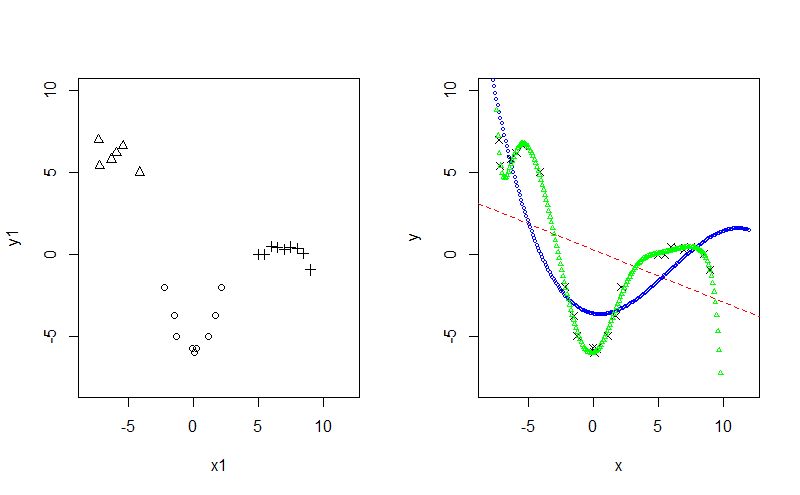Figure 1.3: Polynomial regression

If we look at the three models and see how they handle the extreme left and right points, we see why overfitting can lead to poor results on unseen data. On the right side of the plot, the last series of points (plus signs) have a local linear relationship. However, the polynomial regression line with degree 12 (green triangles) puts too much emphasis on the last point, which is extra noise and the line moves down sharply. This would cause the model to predict extreme negative values for y as x increases, which is not justified if we look at the data. Overfitting is an important issue that we will look at in more detail in later chapters.

By adding square, cube, and more polynomial terms, the model can fit more complex data than if we just used linear functions on the input data. Neural networks use a similar concept, except that, instead of taking polynomial terms of the input variable, they chain multiple regression functions together with nonlinear terms between them.

The following is an example of a neural network architecture. The circles are nodes and the lines are the connections between nodes. If a connection exists between two nodes, the output from the node on the left is the input for the next node. The output value from a node is a matrix operation on the input values to the node and the weights of the node: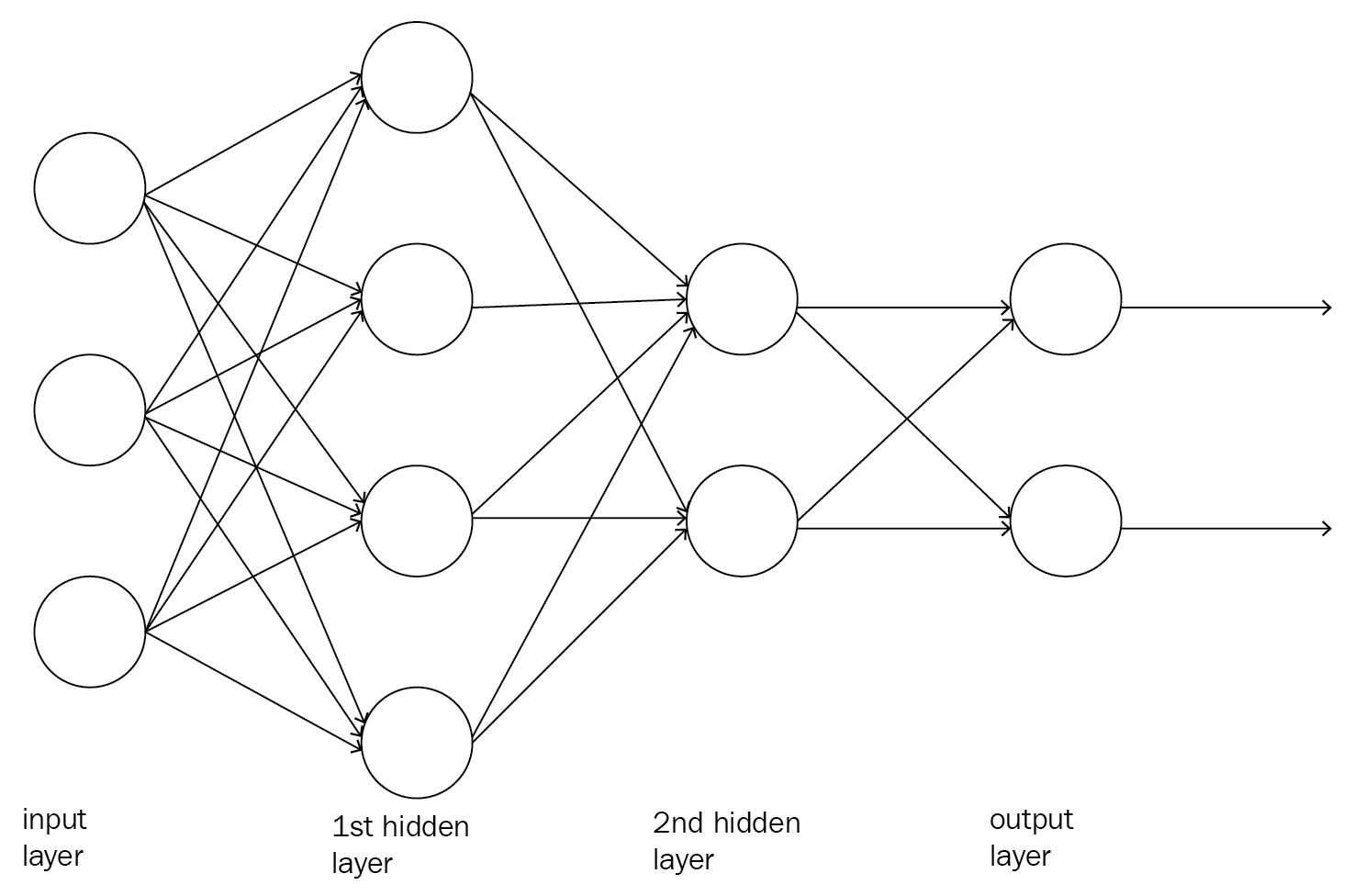Figure 1.4: An example neural network

Before the output values from a node are passed to the next node as input values, a function is applied to the values to change the overall function to a non-linear function. These are known as activation functions and they perform the same role as the polynomial terms.

This idea of creating a machine learning model by combining multiple small functions together is a very common paradigm in machine learning. It is used in random forests, where many small independent decision trees vote for the result. It is also used in boosting algorithms, where the misclassified instances from one function are given more prominence in the next function.

By including many layers of nodes, the neural network model can approximate almost any function. It does make training the model more difficult, so we'll give a brief explanation of how to train a neural network. Each node is assigned a set of random weights initially. For the first pass, these weights are used to calculate and pass (or propagate) values from the input layer to the hidden layers and finally to the output layer. This is known as forward-propagation. Because the weights were set randomly, the final (prediction) values at the output layer will not be accurate compared to the actual values, so we need a method of calculating how different the predicted values are from the actual values. This is calculated using a cost function, which gives a measure of how accurate the model is during training. We then need to adjust the weights in the nodes from the output layer backward to get us nearer to the target values. This is done using backward-propagation; we move from right to left, updating the weights of the nodes in each layer very slightly to get us very slightly closer to the actual values. The cycle of forward-propagation and backward-propagation continues until the error value from the loss function stops getting smaller; this may require hundreds, or thousands of iterations, or epochs.

To update the node weights correctly, we need to know that the change will get us nearer to the target, which is to minimize the result from the cost function. We are able to do this because of a clever trick, we use activation functions that have derivative functions.

If your knowledge of calculus is limited, it can be difficult to get an understanding of derivatives initially. But in simple terms, a function may have a derivative formula that tells us how to change the input of a function so that the output of the function moves in a positive or negative manner. This derivative/formula enables the algorithm to minimize the cost function, which is a measurement of error. In more technical terms, the derivative of the function measures the rate of change in the function as the input changes. If we know the rate of change of a function as the input changes, and more importantly what direction it changes in, then we can use this to get nearer to minimizing that function. An example that you may have seen before is the following diagram: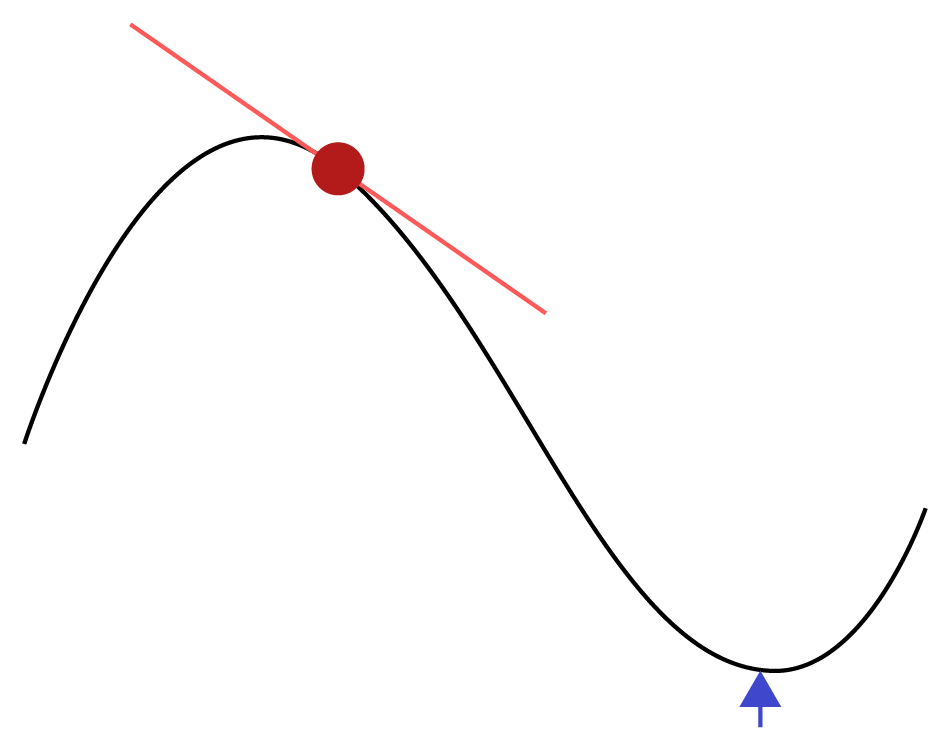Figure 1.5: A function (curved) line and its derivative at a point

In this diagram, the curved line is a mathematical function we want to minimize over y, that is, we want to get to the lowest point (which is marked by the arrow). We are currently at the point in the red circle, and the derivative at that point is the slope of the tangent. The derivative function indicates the direction we need to move in to get there. The derivative value changes as we get nearer the target (the arrow), so we cannot make the move in one big step. Therefore, the algorithm moves in small steps and re-calculates the derivative after each step, but if we choose too small a step, it will take very long to converge (that is, get near the minimum). If we take too big a step, we run the risk of overshooting the minimum value. How big a step you take is known as the learning rate, and it effectively decides how long it takes the algorithm to train.

This might seem a bit abstract, so an analogy should make it somewhat clearer. This analogy may be over-simplified, but it explains derivatives, learning rates, and cost functions. Imagine a simple model of driving a car, where the speed must be set to a value that is suitable for the conditions and the speed limit. The difference between your current speed and the target speed is the error rate and this is calculated using a cost function (just simple subtraction, in this case). To change your speed, you apply the gas pedal to speed up or the brake pedal to slow down. The acceleration/deceleration (that is, the rate of change of the speed) is the derivative of the speed. The amount of force that is applied to the pedals changes how fast the acceleration/deceleration occurs, the force is similar to the learning rate in a machine learning algorithm. It controls how long it takes to get to the target value. If only a small change is applied to the pedals, you will eventually get to your target speed, but it will take much longer. However, you usually don't want to apply maximum force to the pedals, to do so may be dangerous (if you slam on the brakes) or a waste of fuel (if you accelerate too hard). There is a happy medium where you apply the change and get to the target speed safely and quickly.

# Neural networks as a network of memory cells

Another way to consider neural networks is to compare them to how humans think. As their name suggests, neural networks draw inspiration from neural processes and neurons in the mind. Neural networks contain a series of neurons, or nodes, which are interconnected and process input. The neurons have weights that are learned from previous observations (data). The output of a neuron is a function of its input and its weights. The activation of some final neuron(s) is the prediction.

We will consider a hypothetical case where a small part of the brain is responsible for matching basic shapes, such as squares and circles. In this scenario, some neurons at the basic level fire for horizontal lines, another set of neurons fires for vertical lines, and yet another set of neurons fire for curved segments. These neurons feed into higher-order process that combines the input so that it recognizes more complex objects, for example, a square when the horizontal and vertical neurons both are activated simultaneously.

In the following diagram, the input data is represented as squares. These could be pixels in an image. The next layer of hidden neurons consists of neurons that recognize basic features, such as horizontal lines, vertical lines, or curved lines. Finally, the output may be a neuron that is activated by the simultaneous activation of two of the hidden neurons: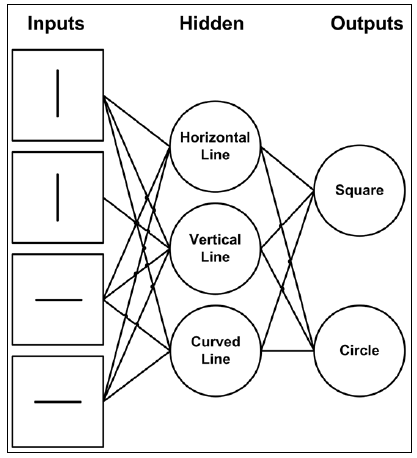Figure 1.6: Neural networks as a network of memory cells

In this example, the first node in the hidden layer is good at matching horizontal lines, while the second node in the hidden layer is good at matching vertical lines. These nodes remember what these objects are. If these nodes combine, more sophisticated objects can be detected. For example, if the hidden layer recognizes horizontal lines and vertical lines, the object is more likely to be a square than a circle. This is similar to how convolutional neural networks work, which we will cover in Chapter 5, Image Classification Using Convolutional Neural Networks.

We have covered the theory behind neural networks very superficially here as we do not want to overwhelm you in the first chapter! In future chapters, we will cover some of these issues in more depth, but in the meantime, if you wish to get a deeper understanding of the theory behind neural networks, the following resources are recommended:

Next, we will turn to a brief introduction to deep neural networks.

# Deep neural networks

A deep neural network (DNN) is a neural network with multiple hidden layers. We cannot achieve good results by just increasing the number of nodes in a neural network with a small number of layers (a shallow neural network). A DNN can fit data more accurately with fewer parameters than a shallow neural network (NN), because more layers (each with fewer neurons) give a more efficient and accurate representation. Using multiple hidden layers allows a more sophisticated build-up from simple elements to more complex ones. In the previous example, we considered a neural network that could recognize basic shapes, such as a circle or a square. In a deep neural network, many circles and squares could be combined to form other, more advanced shapes. A shallow neural network cannot build more advanced shapes from basic pieces. The disadvantage of a DNN is that these models are harder to train and prone to overfitting.

If we consider trying to recognize handwritten text from image data, then the raw data is pixel values from an image. The first layer captures simple shapes, such as lines and curves. The next layer uses these simple shapes and recognizes higher abstractions, such as corners and circles. The second layer does not have to directly learn from the pixels, which are noisy and complex. In contrast, a shallow architecture may require far more parameters, as each hidden neuron would have to be capable of going directly from pixels in the image to the target value. It would also not be able to combine features, so for example, if the image data were in a different location (for example, not centered), it would fail to recognize the text.

One of the challenges in training deep neural networks is how to efficiently learn the weights. The models are complex with a huge number of parameters to train. One of the major advancements in deep learning occurred in 2006, when it was shown that deep belief networks (DBNs) could be trained one layer at a time (See Hinton, G. E., Osindero, S., and Teh, Y. W. (2006)). A DBN is a type of deep neural network with multiple hidden layers and connections between (but not within) layers (that is, a neuron in layer 1 may be connected to a neuron in layer 2, but may not be connected to another neuron in layer 1). The restriction of no connections within a layer allows for much faster training algorithms to be used, such as the contrastive divergence algorithm. Essentially, the DBN can then be trained layer by layer; the first hidden layer is trained and used to transform raw data into hidden neurons, which are then treated as a new set of input in the next hidden layer, and the process is repeated until all the layers have been trained.

The benefits of the realization that DBNs could be trained one layer at a time extend beyond just DBNs. DBNs are sometimes used as a pre-training stage for a deep neural network. This allows comparatively fast, greedy, layer-by­-layer training to be used to provide good initial estimates, which are then refined in the deep neural network using other, less efficient, training algorithms, such as back-propagation.

So far we have primarily focused on feed-forward neural networks, where the results from one layer and neuron feed forward to the next. Before closing this section, two specific kinds of deep neural network that have grown in popularity are worth mentioning. The first is a recurrent neural network (RNN), where neurons send feedback signals to each other. These feedback loops allow RNNs to work well with sequences. An example of an application of RNNs is to automatically generate click-bait, such as Top 10 reasons to visit Los Angeles: #6 will shock you! or One trick great hair salons don't want you to know. RNNs work well for such jobs as they can be seeded from a large initial pool of a few words (even just trending search terms or names) and then predict/generate what the next word should be. This process can be repeated a few times until a short phrase is generated, the click-bait. We will see examples of RNNs in Chapter 7, Natural Language Processing using Deep Learning.

The second type is a convolutional neural network (CNN). CNNs are most commonly used in image-recognition. CNNs work by having each neuron respond to overlapping subregions of an image. The benefits of CNNs are that they require comparatively minimal preprocessing but still do not require too many parameters through weight-sharing (for example, across subregions of an image). This is particularly valuable for images as they are often not consistent. For example, imagine ten different people taking a picture of the same desk. Some may be closer or farther away or at positions resulting in essentially the same image having different heights, widths, and the amount of image captured around the focal object. We will cover CNNs in depth in Chapter 5, Image Classification Using Convolutional Neural Networks.

This description only provides the briefest of overviews as to what deep neural networks are and some of the use cases to which they can be applied. The seminal reference for deep learning is Goodfellow-et-al (2016).

# Some common myths about deep learning

There are many misconceptions, half-truths, and downright misleading opinions on deep learning. Here are some common mis-conceptions regarding deep learning:

• Artificial intelligence means deep learning and replaces all other techniques
• Deep learning requires a PhD-level understanding of mathematics
• Deep learning is hard to train, almost an art form
• Deep learning requires lots of data
• Deep learning has poor interpretability
• Deep learning needs GPUs

The following paragraphs discuss these statements, one by one.

Deep learning is not artificial intelligence and does not replace all other machine learning algorithms. It is only one family of algorithms in machine learning. Despite the hype, deep learning probably accounts for less than 1% of the machine learning projects in production right now. Most of the recommendation engines and online adverts that you encounter when you browse the net are not powered by deep learning. Most models used internally by companies to manage their subscribers, for example churn analysis, are not deep learning models. The models used by credit institutions to decide who gets credit do not use deep learning.

Deep learning does not require a deep understanding of mathematics unless your interest is in researching new deep learning algorithms and specialized architectures. Most practitioners use existing deep learning techniques on their data by taking an existing architecture and modifying it for their work. This does not require a deep mathematical foundation, the mathematics used in deep learning are taught at high school level throughout the world. In fact, we demonstrate this in Chapter 3, Deep Learning Fundamentals, where we build an entire neural network from basic code in less than 70 lines of code!

Training deep learning models is difficult but it is not an art form. It does require practice, but the same problems occur over and over again. Even better, there is often a prescribed fix for that problem, for example, if your model is overfitting, add regularization, if your model is not training well, build a more complex model and/or use data augmentation. We will look at this in more depth in Chapter 6, Tuning and Optimizing Models.

There is a lot of truth to the statement that deep learning requires lots of data. However, you may still be able to apply deep learning to the problem by using a pre-trained network, or creating more training data from existing data (data augmentation). We will look at these in later Chapter 6, Tuning and Optimizing Models and Chapter 11, The Next Level in Deep Learning.

Deep learning models are difficult to interpret. By this, we mean being able to explain how the models came to their decision. This is a problem in many machine learning algorithms, not just deep learning. In machine learning, generally there is an inverse relationship between accuracy and interpretation the more accurate the model needs to be, the less interpretable it is. For some tasks, for example, online advertising, interpretability is not important and there is little cost from being wrong, so the most powerful algorithm is preferred. In some cases, for example, credit scoring, interpretability may be required by law; people could demand an explanation of why they were denied credit. In other cases, such as medical diagnoses, interpretability may be important for a doctor to see why the model decided someone had a disease.

If interpretability is important, some methods can be applied to machine learning models to get an understanding of why they predicted the output for an instance. Some of them work by perturbing the data (that is, making slight changes to it) and trying to find what variables are most influential in the model coming to its decision. One such algorithm is called LIME (Local Interpretable Model-Agnostic Explanations). (Ribeiro, Marco Tulio, Sameer Singh, and Carlos Guestrin. Why should I trust you?: Explaining the predictions of any classifier. Proceedings of the 22nd ACM SIGKDD international conference on knowledge discovery and data mining. ACM, 2016.) This has been implemented in many languages including R; there is a package called lime. We will use this package in Chapter 6, Tuning and Optimizing Models.

Finally, while deep learning models can run on CPUs, the truth is that any real work requires a workstation with a GPU. This does not mean that you need to go out and purchase one, as you can use cloud-computing to train your models. In Chapter 10, Running Deep Learning Models in the Cloud, will look at using AWS, Azure, and Google Cloud to train deep learning models.

# Setting up your R environment

Before you begin your deep learning journey, the first step is to install R, which is available at https://cran.r-project.org/. When you download R and use it, only a few core packages are installed by default, but new packages can be added by selecting from a menu option or by a single line of code. We will not go into detail on how to install R or how to add packages, we assume that most readers are proficient in these skills. A good integrated development environment (IDE) for working with R is essential. By far the most popular IDE, and my recommendation, is RStudio, which can be downloaded from https://www.rstudio.com/. Another option is Emacs. An advantage of both Emacs and RStudio is that they are available on all major platforms (Windows, macOS, and Linux), so even if you switch computers, you can have a consistent IDE experience. The following is a screenshot of the RStudio IDE: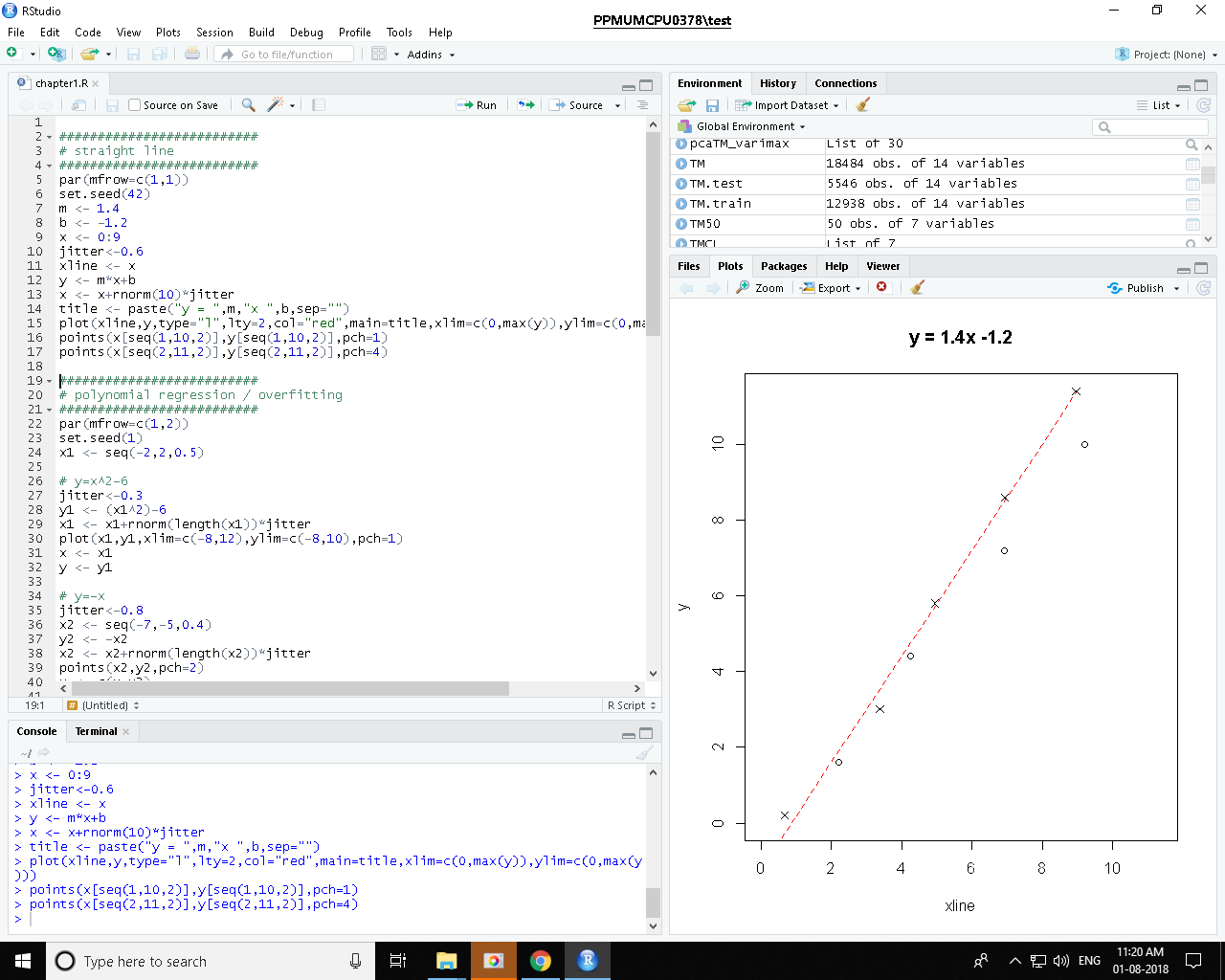Figure 1.7 RStudio IDE

Using RStudio is a major improvement over the R GUI in Windows. There are a number of panes in RStudio that provide different perspectives on your work. The top-left pane shows the code, the bottom-left pane shows the console (results of running the code). The top-right pane shows the list of variables and their current values, the bottom-right pane shows the plots created by the code. All of these panes have further tabs to explore further perspectives.

As well as an IDE, RStudio (the company) have either developed or heavily supported other tools and packages for the R environment. We will use some of these tools, including the R Markdown and R Shiny applications. R Markdown is similar to Jupyter or IPython notebooks; it allows you to combine code, output (for example, plots), and documentation in one script. R Markdown was used to create sections of this book where code and descriptive text are interwoven. R Markdown is a very good tool to ensure that your data science experiments are documented correctly. By embedding the documentation within the analysis, they are more likely to stay synchronized. R Markdown can output to HTML, Word, or PDF. The following is an example of an R Markdown script on the left and the output on the right: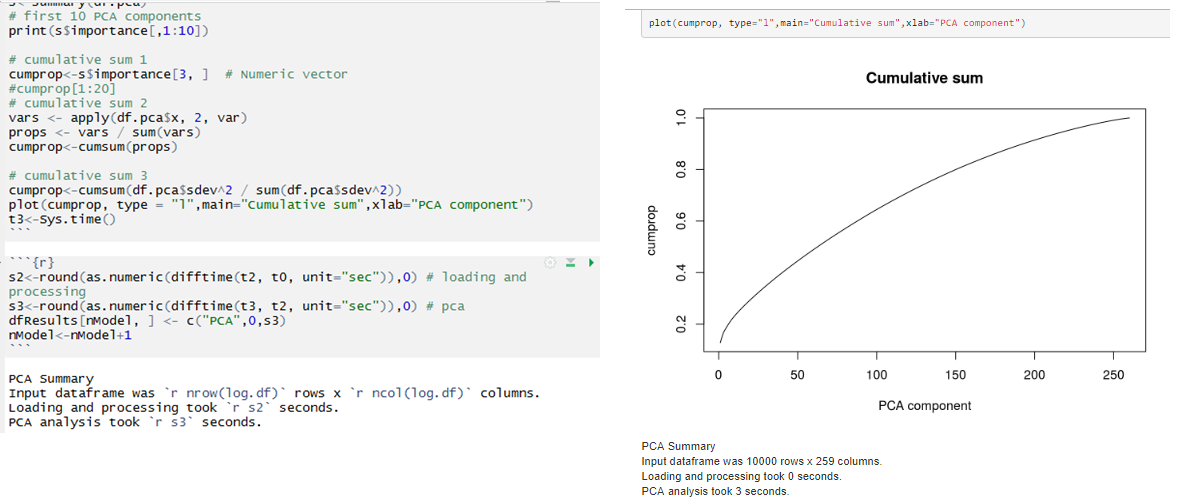Figure 1.8: R Markdown example; on the left is a mixture of R code and text information. The output on the right is HTML generated from the source script.

We will also use R Shiny to create web applications using R. This is an excellent method to create interactive applications to demonstrate key functionality. The following screenshot is an example of an R Shiny web application, which we will see in Chapter 5, Image Classification Using Convolutional Neural Networks: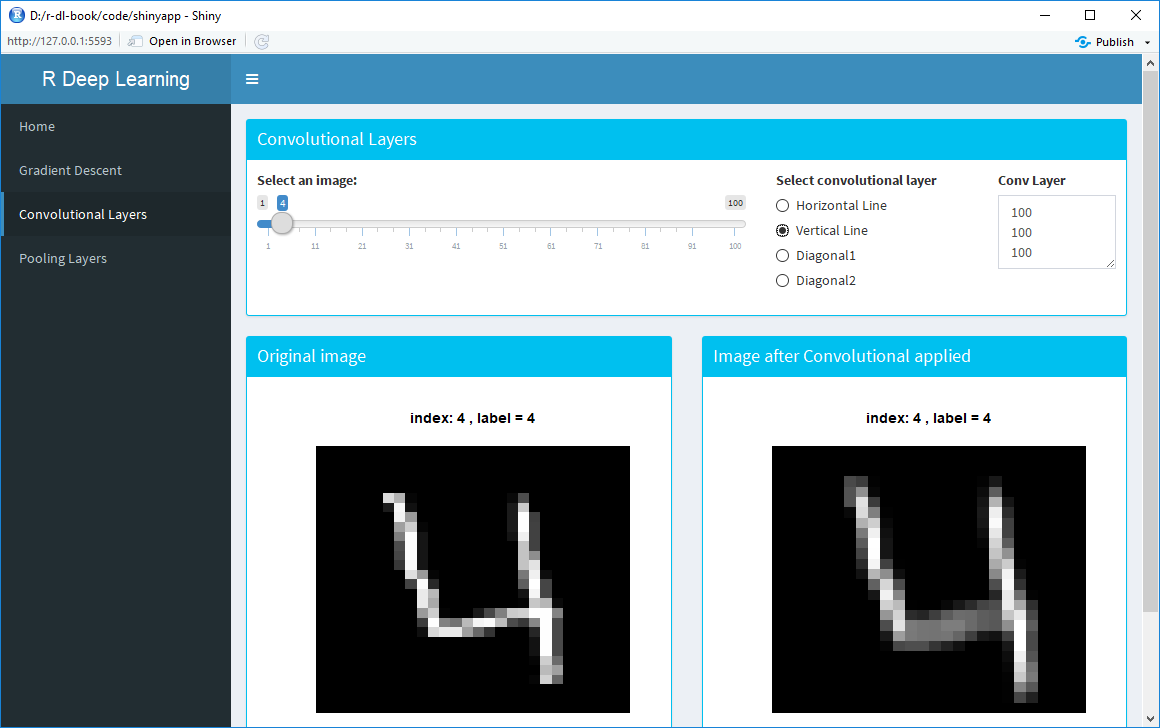Figure 1.9: An example of an R Shiny web application

Once you have R installed, you can look at adding packages that can fit basic neural networks. The nnet package is one package and it can fit feed-forward neural networks with one hidden layer, such as the one shown in Figure 1.6. For more details on the nnet package, see Venables, W. N. and Ripley, B. D. (2002). The neuralnet package fits neural networks with multiple hidden layers and can train them using back-propagation. It also allows custom error and neuron-activation functions. We will also use the RSNNS package, which is an R wrapper of the Stuttgart Neural Network Simulator (SNNS). The SNNS was originally written in C, but was ported to C++. The RSNNS package makes many model components from SNNS available, making it possible to train a wide variety of models. For more details on the RSNNS package, see Bergmeir, C., and Benitez, J. M. (2012). We will see examples of how to use these models in Chapter 2, Training a Prediction Model.

The deepnet package provides a number of tools for deep learning in R. Specifically, it can train RBMs and use these as part of DBNs to generate initial values to train deep neural networks. The deepnet package also allows for different activation functions, and the use of dropout for regularization.

# Deep learning frameworks for R

There are a number of R packages available for neural networks, but few options for deep learning. When the first edition of this book came out, it used the deep learning functions in h2o (https://www.h2o.ai/). This is an excellent, general machine learning framework written in Java, and has an API that allows you to use it from R. I recommend you look at it, especially for large datasets. However, most deep learning practitioners had a preference preferred other deep learning libraries, such as TensorFlow, CNTK, and MXNet, which were not supported in R when the first edition of this book was written. Today, there is a good choice of deep learning libraries that are supported in R—MXNet and Keras. Keras is actually a frontend abstraction for other deep learning libraries, and can use TensorFlow in the background. We will use MXNet, Keras, and TensorFlow in this book.

# MXNet

MXNet is a deep learning library developed by Amazon. It can run on CPUs and GPUs. For this chapter, running on CPUs will suffice.

Apache MXNet is a flexible and scalable deep learning framework that supports convolutional neural networks (CNNs) and long short-term memory networks (LSTMs). It can be distributed across multiple processors/machines and achieves almost linear scale on multiple GPUs/CPUs. It is easy to install on R and it supports a good range of deep learning functionality for R. It is an excellent choice for writing our first deep learning model for image-classification.

MXNet originated at Carnegie Mellon University and is heavily supported by Amazon; they chose it as their default deep learning library in 2016. In 2017, MXNet was accepted as the Apache Incubator project, ensuring that it would remain open source software. It has a higher-level programming model similar to Keras, but the reported performance is better. MXNet is very scalable as additional GPUs are added.

To install the MXNet package for Windows, run the following code from an R session:

`cran <- getOption("repos")cran["dmlc"] <- "https://apache-mxnet.s3-accelerate.dualstack.amazonaws.com/R/CRAN"options(repos = cran)install.packages("mxnet")`

This installs the CPU version; for the GPU version, you need to change the second line to:

`cran["dmlc"] <- "https://apache-mxnet.s3-accelerate.dualstack.amazonaws.com/R/CRAN/GPU/cu92"`

You have to change cu92 to cu80, cu90 or cu91 based on the version of CUDA installed on your machine. For other operating systems (and in case the this does not work, as things change very fast in deep learning), you can get further instructions at https://mxnet.incubator.apache.org/install/index.html.

# Keras

Keras is a high-level, open source, deep learning framework created by Francois Chollet from Google that emphasizes iterative and fast development; it is generally regarded as one of the best options to use to learn deep learning. Keras has a choice of backend lower-level frameworks: TensorFlow, Theano, or CNTK, but it is most commonly used with TensorFlow. Keras models can be deployed on practically any environment, for example, a web server, iOS, Android, a browser, or the Raspberry Pi.

To learn more about Keras, go to https://keras.io/. To learn more about using Keras in R, go to https://keras.rstudio.com; this link will also has more examples of R and Keras, as well as a handy Keras cheat sheet that gives a thorough reference to all of the functionality of the R Keras package. To install the keras package for R, run the following code:

`devtools::install_github("rstudio/keras")library(keras)install_keras()`

This will install the CPU-based package of Keras and TensorFlow. If your machine has a suitable GPU, you can refer to the documentation for install_keras() to find out how to install it.

# Do I need a GPU (and what is it, anyway)?

Probably the two biggest reasons for the exponential growth in deep learning are:

• The ability to accumulate, store, and process large datasets of all types
• The ability to use GPUs to train deep learning models

So what exactly are GPUs and why are they so important to deep learning? Probably the best place to start is by actually looking at the CPU and why this is not optimal for training deep learning models. The CPU in a modern PC is one of the pinnacles of human design and engineering. Even the chip in a mobile phone is more powerful now than the entire computer systems of the first space shuttles. However, because they are designed to be good at all tasks, they may not be the best option for niche tasks. One such task is high-end graphics.

If we take a step back to the mid-1990s, most games were 2D, for example, platform games where the character in the game jumps between platforms and/or avoids obstacles. Today, almost all computer games utilize 3D space. Modern consoles and PCs have co-processors that take the load of modelling 3D space onto a 2D screen. These co-processors are known as GPUs.

GPUs are actually far simpler than CPUs. They are built to just do one task: massively parallel matrix operations. CPUs and GPUs both have cores, where the actual computation takes place. A PC with an Intel i7 CPU has four physical cores and eight virtual cores by using Hyper Threading. The NVIDIA TITAN Xp GPU card has 3,840 CUDA® cores. These cores are not directly comparable; a core in a CPU is much more powerful than a core in a GPU. But if the workload requires a large amount of matrix operations that can be done independently, a chip with lots of simple cores is much quicker.

Before deep learning was even a concept, researchers in neural networks realized that doing high-end graphics and training neural networks both involved workloads: large amounts of matrix multiplication that could be done in parallel. They realized that training the models on the GPU rather than the CPU would allow them to create much more complicated models.

Today, all deep learning frameworks run on GPUs as well as CPUs. In fact, if you want to train models from scratch and/or have a large amount of data, you almost certainly need a GPU. The GPU must be an NVIDIA GPU and you also need to install the CUDA® Toolkit, NVIDIA drivers, and cuDNN. These allow you to interface with the GPU and hijack its use from a graphics card to a maths co-processor. Installing these is not always easy, you have to ensure that the versions of CUDA, cuDNN and the deep learning libraries you use are compatible. Some people advise you need to use Unix rather than Windows, but support on Windows has improved greatly. This code on this book was developed on a Windows workstation. Forget about using a macOS, because they don't support NVIDIA cards.

That was the bad news. The good news is that you can learn everything about deep learning if you don't have a suitable GPU. The examples in the early chapters of this book will run perfectly fine on a modern PC. When we need to scale up, the book will explain how to use cloud resources, such as AWS and Google Cloud, to train large deep learning models.

# Setting up reproducible results

Software for data science is advancing and changing rapidly. Although this is wonderful for progress, it can make reproducing someone else's results a challenge. Even your own code may not work when you go back to it a few months later. This is one of the biggest issues in scientific research today, across all fields, not just artificial intelligence and machine learning. If you work in research or academia and you want to publish your results in scientific journals, this is something you need to be concerned about. The first edition of this book partially addressed this problem by using the R checkpoint package provided by Revolution Analytics. This makes a record of what versions of software were used and ensures there is a snapshot of them available.

For the second edition, we will not use this package for a number of reasons:

• Most readers are probably not publishing their work and are more interested in other concerns (maximizing accuracy, interpretability, and so on).
• Deep learning requires large datasets. When you have a large amount of data, it should mean that, while we may not get precisely the same result each time, it will be very close (fractions of percentages).
• In production systems, there is more to reproducibility than software. You also have to consider data pipelines and random seed-generation.
• In order to ensure reproducibility, the libraries used must stay frozen. New versions of deep learning APIs are released constantly and may contain enhancements. If we limited ourselves to old versions, we would get poor results.

If you are interested in learning more about the checkpoint package, you can read the online vignette for the package at https://cran.r-project.org/web/packages/checkpoint/vignettes/checkpoint.html.

This book was written using R version 3.5 on Windows 10 Professional x64, which is the latest version of R at the time of writing. The code was run on a machine with an Intel i5 processor and 32 GB RAM; it should run on an Intel i3 processor with 8 GB RAM.

You can download the example code files for this book from your account at http://www.packtpub.com/. If you purchased this book elsewhere, you can visit http://www.packtpub.com/support and register to have the files emailed directly to you.

2. Hover the mouse pointer on the SUPPORT tab at the top.
4. Enter the name of the book in the Search box.
5. Select the book for which you're looking to download the code files.
6. Choose from the drop-down menu where you purchased this book from.

• WinRAR I 7-Zip for Windows
• Zipeg I iZip I UnRarX for Mac
• 7-Zip I PeaZip for Linux

# Summary

This chapter presented a brief introduction to neural networks and deep neural networks. Using multiple hidden layers, deep neural networks have been a revolution in machine learning. They consistently outperform other machine learning tasks, especially in areas such as computer vision, natural-language processing, and speech-recognition.

The chapter also looked at some of the theory behind neural networks, the difference between shallow neural networks and deep neural networks, and some of the misconceptions that currently exist concerning deep learning.

We closed this chapter with a discussion on how to set up R and the importance of using a GUI (RStudio). This section discussed the deep learning libraries available in R (MXNet, Keras, and TensorFlow), GPUs, and reproducibility.

In the next chapter, we will begin to train neural networks and generate our own predictions.